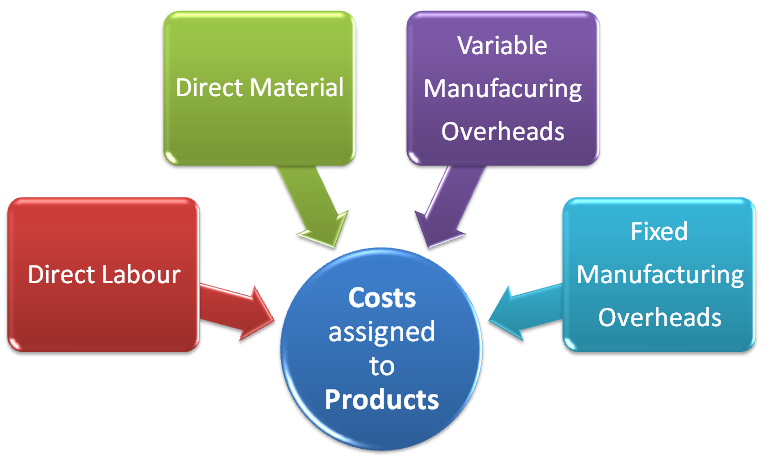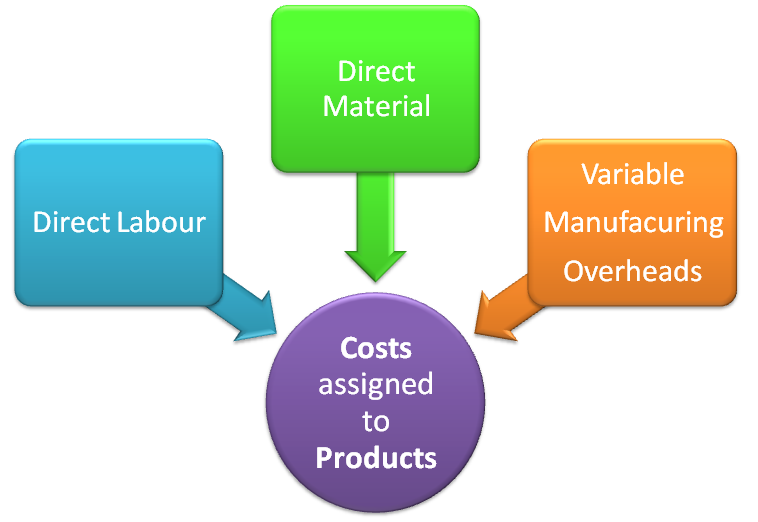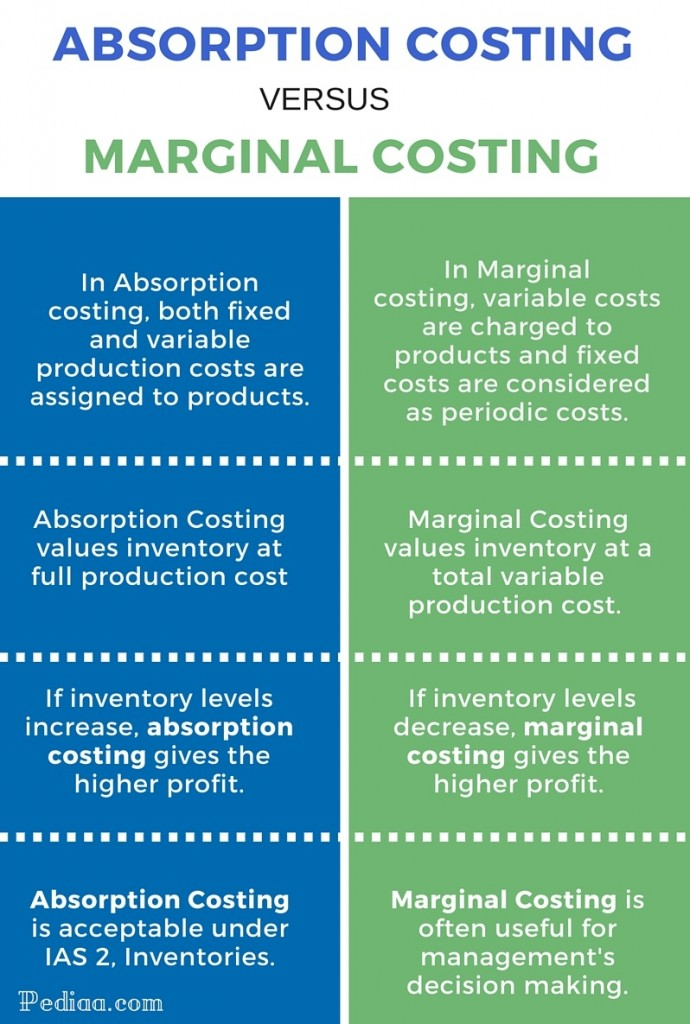# Difference between Absorption Costing and Marginal Costing

## Main Difference – Absorption Costing vs. Marginal Costing

Marginal costing and absorption costing are two different approaches dealing with fixed production overheads. In other words, this involves determining whether or not to include fixed overheads in decision making such as inventory valuation, pricing, etc. Absorption costing is a method of costing a product in which all fixed and variable production costs are apportioned to products. This method ensures that costs incurred are recovered from the selling price of a product. Marginal costing is an accounting system in which variable costs are charged to products and fixed costs are considered as periodic costs. The main difference between absorption costing and marginal costing lies in how the two techniques treat fixed production overheads. Under marginal costing, fixed manufacturing overhead costs are not allocated to products. This is contrasted with absorption costing, where fixed manufacturing overheads are absorbed by products. Absorption costing is a procedure of tracing both variable costs and fixed costs of production to the product whereas marginal costing traces only variable costs of production to the product while fixed costs of production are considered periodic expenses.

## What is Absorption Costing

Absorption costing is a method of calculating the full cost of a product. As a result, absorption costing is also known as full costing. Under absorption costing, the entire cost of production is apportioned to products. These costs could be direct costs or indirect costs (variable and fixed overheads). Fixed overheads are usually applied based on a predetermined overhead absorption rate. One or more overhead absorption rates could be employed.

Costs assigned to products under absorption costing are as follows;

• Direct material: Materials included in a finished product
• Direct labour: Labour cost required to construct a product
• Variable manufacturing overheads: Cost of operating a manufacturing facility, which vary with production volume i.e. electricity for production equipment
• Fixed manufacturing overheads: Cost of operating a manufacturing facility, which do not vary with production volume i.e. rent

Absorption costing ensures that all incurred costs are recovered from selling price of a good or service. Opening and closing inventory are valued at full production cost under absorption costing.

Let’s consider the below example.

A factory produces product ‘A’ which sells at \$50,000 each. The direct cost of manufacturing a unit of the product is \$10,000 for materials and \$20,000 for direct labour. The fixed overhead expense in one year is \$10 million. Direct labour hours related to each unit of product are 100 hours.  The capacity of labour in one year is 100,000 hours.

If overheads could be allocated based on labour hours, an overhead absorption rate for product A could be calculated as follows;

Fixed overhead expense per year                              =             \$10,000,000

Total direct labour hours per year                           =             100,000

Fixed overhead per direct labour hour                    =             \$100

Direct labour hours per unit                                      =             100

Fixed overhead per unit                                              =             \$10,000

Total cost allocated to product A using absorption costing is the addition of direct material, direct labour and fixed overhead cost which is \$10,000 + \$20,000 + \$10,000 = \$40,000 per unit of A.

As each product sells at \$50,000, absorption costing system calculates a profit of \$10,000 on each unit sold of product A.## What is Marginal Costing

When an additional unit of a product is manufactured, the extra cost incurred is the variable cost of production. Fixed costs are unaffected and no extra fixed cost is incurred when output is increased. The marginal cost of a product is its variable cost which is usually direct labour, direct material, direct expenses and variable production overheads. Marginal costing is used to understand the impact of variable cost on volume of production. As a result, this technique is also known as variable costing or direct costing.

Marginal costing is the accounting system in which variable costs are charged to products and fixed costs are considered as periodic costs and written off in full against contribution. Under marginal costing, the contribution is the foundation to know the profitability of a product. The contribution is equal to the selling price of a product less marginal cost. Fixed cost is recovered from contribution. Further, opening and closing inventory are valued at marginal (variable) cost.

Marginal costing is the principal costing technique used in decision making. The main reason for this is, the marginal costing approach allows management to be focused on changes resulting from the decision in concern.

If we consider the same example as above, marginal cost per unit of product A would be the addition of direct material and direct labour which is \$10,000 + \$20,000 = \$30,000 per unit of A. As each product sells at \$50,000, marginal costing system calculates a contribution of \$20,000 on each unit sold of product A. Fixed overhead of \$10 million will be treated as a periodic cost, not as a cost related to the product.## Difference Between Absorption Costing and Marginal Costing

As we have now understood the two terms separately, we will compare the two in order to find other differences between Absorption Costing and Marginal Costing.

### Definition

Absorption costing is a method of costing a product in which all fixed and variable production costs are apportioned to products.

Marginal costing is an accounting system in which variable costs are charged to products and fixed costs are considered as periodic costs.

### Inventory Valuation

Absorption Costing values inventory at full production cost. Fixed cost relating to closing stock is carried forward to the next year. Similarly, fixed cost relating to an opening stock is charged to the current year instead of the previous year. Thus, under absorption costing, all fixed cost is not charged against revenue of the year in which they are incurred.

Marginal Costing values inventory at a total variable production cost. Therefore, there is no chance of carrying forward unreasonable fixed overheads from one accounting period to the next. However, under marginal costing, the value of inventory is understated.

### Effect on Profit

As inventory values are different under absorption and marginal costing, profits too differ under two techniques.

1. If inventory levels increase, absorption costing gives the higher profit.

This is because fixed overheads held in closing inventory are carried forward to the next accounting period instead of being written off in the current accounting period.

2. If inventory levels decrease, marginal costing gives the higher profit.

This is because the fixed overhead brought forward in opening inventory is released, thereby increasing the cost of sales and reducing profits.

• If inventory levels are constant, both methods give the same profit.

### Treatment of Fixed Cost – Outcome

Absorption Costing includes fixed production overheads in inventory values. However, fixed overheads cannot be absorbed exactly due to difficulties in forecasting costs and volume of output. Therefore, there is the possibility that overheads could be over or under absorbed. Overhead is over-absorbed when the amount allocated to a product is higher than the actual amount and it is under absorbed when the amount allocated to a product is lower than the actual amount.

In Marginal Costing, fixed production overheads are not shared out among units of production. Actual fixed overhead incurred is charged against contribution as a periodic cost.

### Usefulness of the Technique

Absorption Costing is more complex to operate and it does not provide any useful information for decision making like marginal costing. Cost data produced under absorption costing is not very useful for decision making because product cost includes fixed overhead obscuring cost-volume-profit relationship. However, absorption costing is required for external financial reporting and income tax reporting.

Marginal Costing does not allocate fixed manufacturing overheads to a product. As a result, marginal costing could be more useful for incremental pricing decisions where a company is more concerned about additional cost required to build the next unit. Identification of variable costs and contribution enables management to use cost information more easily for decision making.

### Presentation in Financial Statements

Absorption Costing is acceptable under IAS 2, Inventories. Thus, absorption costing is required for external financial reporting and income tax reporting.

Marginal Costing is often useful for management’s decision making. Exclusion of fixed cost from inventory affects profit. Therefore, true and fair view of financial statements may not be clearly transparent under marginal costing.

### Summary – Absorption Costing vs Marginal Costing

In this article, we have attempted to understand the terms absorption costing and marginal costing followed by a comparison to highlight key differences between them. The basic difference between Absorption Costing and Marginal Costing lies in how fixed overhead cost is treated in management decisions of valuation of inventory and pricing. In absorption costing, fixed cost is included in both value of inventory and cost of the product when making the pricing decision whereas marginal costing avoids fixed overheads in both decisions.References:

ACCAPEDIA – Kaplan.” Kaplan Financial Knowledge Bank. N.p., n.d. Web. 30 Oct. 2015.

Criticism of Marginal Costing | Limitations of Absorption …” tutorsonnet.com.N.p., n.d. Web. 30 Oct. 2015.

Cost Accounting | Case Study Solution | Case Study Analysis.” Accounting Blog. N.p., n.d. Web. 30 Oct. 2015.